基于ANSYS软件对SHPB实验过程的数值模拟研究Numerical Simulation of SHPB Experimental Process Based on ANSYS Software

• 全文下载: PDF(930KB)    PP.39-46   DOI: 10.12677/IJM.2019.81005
• 下载量: 116  浏览量: 266

In order to better study the stress propagation problem in the numerical simulation of the split Hopkinson pressure bar (SHPB) experiment, a numerical three-dimensional model of SHPB is es-tablished based on ANSYS/LS-DYNA software, and the mechanical properties of different speed bullets are simulated during the SHPB experiment. The results are calculated and compared to a standard graph. The results show that the ANSYS/LS-DYNA software can effectively simulate the SHPB experiment and obtain an effective stress-strain curve of the test piece. The accuracy of the result is significantly improved by the fine mesh method.

1. 引言

2. SHPB实验

2.1. 实验概况

2.2. SHPB实验的两个假定

1) 一维应力波假定：假设应力脉冲在杆中是无畸变的线弹性应力波。由于入射波的波长和入射杆的直径相比较，要大许多。因此试验中可忽略杆的横向振动，即试样满足一维应力的条件。此时我们可以忽略杆中应力波传播的弥散效应，即只存在轴向应力，我们可以认为，在波导杆中间部位的信息，可以代替波导杆与试件接触处的信息，根据一维应力波理论从而求出试件的应力–应变曲线。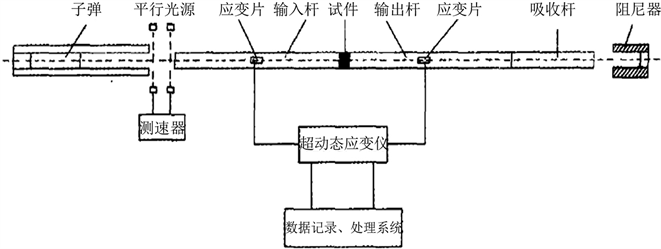Figure 1. SHPB experimental device

${u}_{1}\left(t\right)={C}_{0}{\int }_{0}^{t}\left[{\epsilon }_{i}\left(t\right)-{\epsilon }_{r}\left(t\right)\right]\text{d}t$ (2.1)

${u}_{2}\left(t\right)={C}_{0}{\int }_{0}^{t}{\epsilon }_{t}\left(t\right)\text{d}t$ (2.2)

${\epsilon }_{t}=\frac{{u}_{1}\left(t\right)-{u}_{2}\left(t\right)}{{L}_{0}}=\frac{{C}_{0}}{{L}_{0}}{\int }_{0}^{t}\left[{\epsilon }_{i}\left(t\right)-{\epsilon }_{r}\left(t\right)-{\epsilon }_{t}\left(t\right)\right]\text{d}t$ (2.3)

$\stackrel{˙}{\epsilon }\left(t\right)=\frac{{C}_{0}}{{L}_{0}}\left[{\epsilon }_{i}\left(t\right)-{\epsilon }_{r}\left(t\right)-{\epsilon }_{t}\left(t\right)\right]$ (2.4)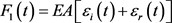(2.5)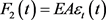(2.6)

$\sigma \left(t\right)=\frac{{F}_{1}\left(t\right)+{F}_{2}\left(t\right)}{2{A}_{0}}=\frac{EA}{2{A}_{0}}\epsilon \left(t\right)$ (2.7)

${A}_{0}$ 为试件的截面面积， ${F}_{1}\left(t\right)$ 入射方轴力， ${F}_{2}\left(t\right)$ 透射方轴力，E试件弹性模量， ${\epsilon }_{}\left(t\right)$ 试件平均应变。

2) 均匀性假定：我们认为，当在界面处产生反射波以及在界面处产生透射波，分别向入射杆和透射杆传播的过程中，应力波也同时在试件内部在两个界面中间不停的往返传播。可以考虑当试件无限小时，试件内部沿杆方向的应力/应变分布将逐渐趋于均匀化。这样，在实验过程中可以忽略试件的应力波效应。这就是SHPB实验技术能够成立的第二个假定——均匀性假定。(2.8)

$\begin{array}{l}\sigma \left(t\right)=\frac{EA}{{A}_{0}}{\epsilon }_{t}\left(t\right)\\ \epsilon \left(t\right)=\frac{2{C}_{0}}{{L}_{0}}{\int }_{0}^{t}\left[{\epsilon }_{i}\left(t\right)-{\epsilon }_{t}\left(t\right)\right]\text{d}t\\ \stackrel{˙}{\epsilon }\left(t\right)=\frac{2{C}_{0}}{{L}_{0}}\left[{\epsilon }_{i}\left(t\right)-{\epsilon }_{t}\left(t\right)\right]\end{array}$ (2.9)

3. SHPB实验过程的数值模拟

3.1. 有限元模型Table 1. Device and specimen dimensionsTable 2. Bullets, incident rods, transmission rod materialsTable 3. Materials of the test piece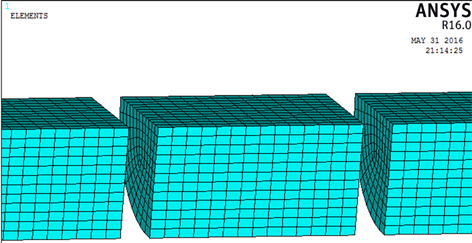Figure 2. 1/4 model meshing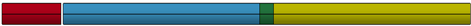Figure 3. SPHB rod numerical model diagram

3.2. 模拟结果对比与分析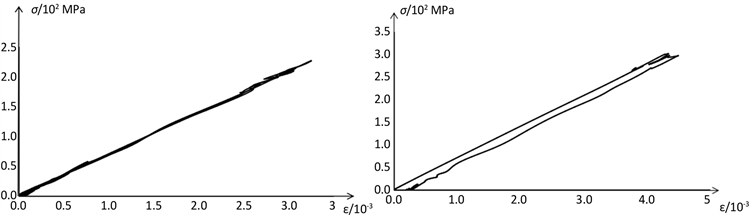(a) 10 m/s (b) 15 m/s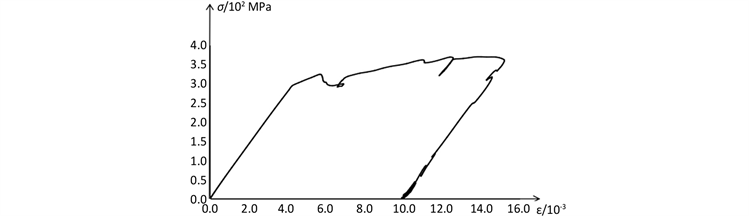(c) 20 m/s

Figure 4. Stress-strain curve of the test piece

3.3. 网格划分对应力波传播过程的影响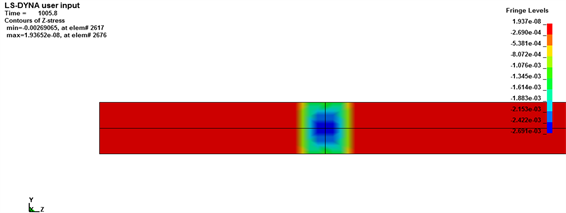(a)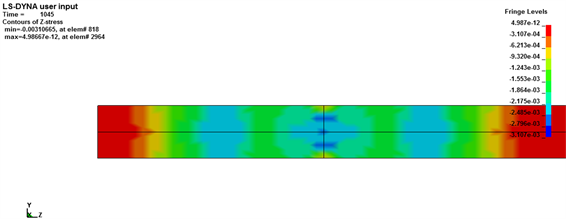(b)

Figure 5. Stress diagram of unit cell meshing; (a) After contact 8 μs; (b) After contact 45 μs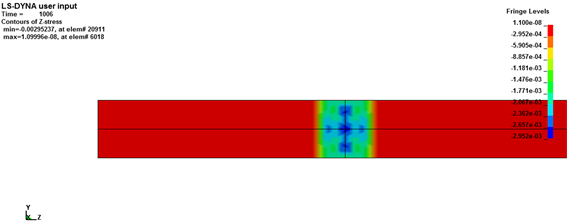(a)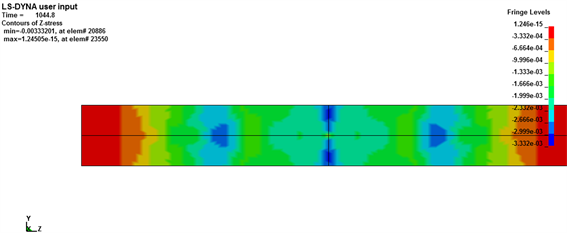(b)

Figure 6. Stress diagram for 1/2 unit cell meshing; (a) After contact 8 μs; (b) After contact 45 μs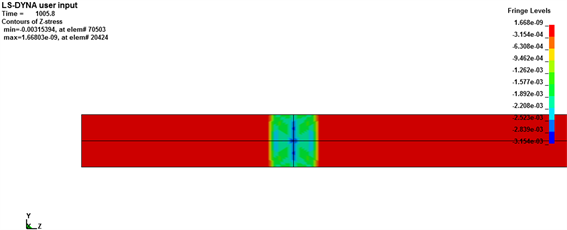(a)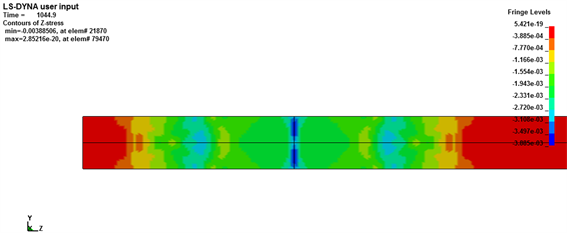(b)

Figure 7. Stress diagram for 1/3 unit cell meshing; (a) After contact 8 μs; (b) After contact 45 μs

4. 结论

1) 数值模拟可以体现实验过程中试件的力学行为，无论在弹性变形阶段还是在塑性变形阶段均一致。

2) 网格划分的大小对应力波传导有明显的影响，网格划分越小，更加能够减少应力波的弥散效应，提高结果的准确性，这种区别随着接触时间的增加有加大趋势。

3) 建议使用1/3单元网格划分构件，经实验进一步的细化网格，准确度提高不明显，但运算时间会加长。

  李为民, 许金余, 沈刘军, 等. SHPB应力均匀及恒应变率加载试验技术研究[J]. 振动与冲击, 2008, 27(2): 115-182.  冯明德, 彭艳菊, 刘永强, 等. SHPB实验技术研究[J]. 地球物理学进展, 2006, 21(1): 273-278.  陶俊林. SHPB实验技术若干问题研究[D]: [博士学位论文]. 北京: 中国工程物理研究院, 2005.  张柱, 晋艳娟. 混凝土材料的SHPB实验技术研究[J]. 太原科技大学学报, 2012, 33(2): 118-122.  王鲁明, 赵坚, 华安增, 等. 脆性材料SHPB实验技术的研究[J]. 岩石力学与工程学报, 2003, 22(11): 1798-1802.  王斌, 李夕兵, 尹土兵, 等. 饱水岩石动态强度的SHPB试验研究[J]. 岩石力学与工程学报, 2010, 29(5): 1003-1009.  平琦, 马芹永, 张经双, 等. 高应变率下砂岩动态拉伸性能SHPB试验与分析[J]. 岩石力学与工程学报, 2012, 31(增1): 3363-3369.  周子龙, 李夕兵, 岩小明. 岩石SHPB测试中试样恒应变率变形的加载条件[J]. 岩石力学与工程学报, 2009, 28(12): 2445-2452.  刘石, 许金余, 刘军忠, 等. 绢云母石英片岩和砂岩的SHPB试验研究[J]. 岩石力学与工程学报, 2011, 30(9): 1864-1871.  宋力, 胡时胜. SHPB测试中的均匀性问题及恒应变率[J]. 爆炸与冲击, 2005, 25(3): 207-216.  宋力, 胡时胜. 一种用于软材料测试的改进SHPB装置[J]. 实验力学, 2004, 19(4): 448-452.  宋力, 胡时胜. SHPB数据处理中的二波法和的三波法[J]. 爆炸与冲击, 2005, 25(4): 368-373.